Power supplies can be classified into two broad categories, one is the AC Power supply and the other is DC power supply. As we know only AC power can be generated and since it is more economical we use AC for transmission and thus most of the electrical machines/devices runs on AC power. But the standard Voltage and Frequency supplied from the Generating stations might not be good enough to drive certain Industrial machines. In those cases we employ converters and inverters to convert one form of power supply into another form such as into a different voltage rating, current rating or frequency rating. A Cycloconveter is one such converter which converts AC power in one frequency into AC power of an adjustable frequency. In this article we will learn more about these Cycloconverters their types, working and applications.

What is Cycloconverter?

The standard definition for Cycloconverters from Wikipedia goes as follows “A cycloconverter (CCV) or a cycloinverter converts a constant voltage, constant frequency AC waveform to another AC waveform of a lower frequency by synthesizing the output waveform from segments of the AC supply without an intermediate DC link”

One particular property of Cycloconverters is that it does not use a DC link in the conversion process thus making it highly efficient. The conversion is done by using power electronic switches likes   Thyristors and switching them in a logical manner. Normally these Thyristors will be separated into two half, the positive half and the negative half. Each half will be made to conduct by turning them during each half cycle of the AC form thus enabling bi-directional power flow. For now imagine Cycloconverters as a black box which take in a fixed Voltage fixed Frequency AC power as input and provides a Variable frequency, variable Voltage as output as shown in the illustration below.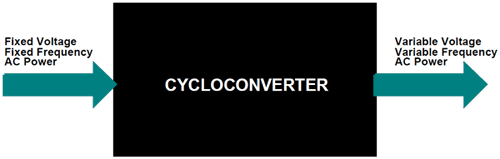We will learn what could possibly be going on inside this black box as we go through the article.

Why do we need Cycloconverters?

Okay, now we know that Cycloconveters convert AC power of fixed frequency to AC Power of variable Frequency. But why do we need to do that? What is the advantage of having an AC power supply which variable Frequency?

The answer to this question is Speed Control. Cycloconveters are extensively used for driving large motors like the one used in Rolling mills, Ball mills Cement kils etc. The out frequency of a Cycloconverters can be reduced upto to zero which helps us to start very large motors with full load at minimum speed and then gradually increase the speed of the motor by increasing the output Frequency. Before the invention of Cycloconverters, these large motors has to be unloaded completely and then after starting the motor it has to be loaded gradually which results in time and man power consumption.

Types of Cycloconveters:

Based on the output frequency and number of phase in the input AC power source the Cycloconverters can be classified as below

1. Step-Up Cycloconverters

2. Ste-Down Cycloconverters

1. Single-Phase to Single-Phase Cycloconverter
2. Three-Phase to Single-Phase Cycloconverter
3. Three-Phase to Three-Phase Cycloconverter

Step-Up Cycloconverters: Step-Up CCV, as the name suggests this type of CCV provide output frequency greater than that of input frequency. But it is not widely used since it not have much particle application. Most application will require a frequency less than 50Hz which is the default frequency here in India. Also Step-Up CCV will require forced commutation which increases the complexity of the circuit.

Step-Down Cycloconverters: Step-Down CCV, as you might have already guessed it well.. just provides an output frequency which is lesser then the input frequency. These are most commonly used and work with help of natural commutation hence comparatively easy to build and operate. The Step-Down CCV is further classified into three types as shown below we will look into each of these types in detail in this article.

Basic Principle behind Cycloconverters:

Although there are three different types of Cycloconverters, the working of them are very similar except for the number of power electronic switches present in the circuit. For instance a single phase to Single Phase CCV will have only 6 power electronic switches (SCR’s) while an Three Phase CCV might have upto 32 switches.The bare minimum for a Cycloconverter is shown above. It will have a Switching circuit on either side of the Load, one circuit will function during the positive half cycle of the AC power source and the other circuit will function during the negative half cycle. Normally the switching circuit will be demonstrated using SCR as power electronic device, but in modern CCV you can find the SCR’s being replaced by IGBT’s and sometimes even MOSFETS.

The switching circuits will also need a control circuit, which instructs the Power electronic device when to conduct and when to turn off. This control circuit will normally be a Microcontroller and might also have a feedback from the output to form a closed loop system .The user can control the value of output frequency by adjusting the parameters in the control circuit.The diodes in the above diagram are used to represent the direction of flow of current. The positive switching circuit always sources current into the load and the negative switching circuit always sinks current from the load.

Single Phase to Single Phase Cycloconverters:

The Single Phase to Single Phase CCV is very rarely used, but to understand the operation of a CCV it should be first studied so that we can understand the Three Phase CCV. The Single Phase to Single Phase CCV has two pairs of full wave rectifier circuit, each consisting of four SCR. One set is placed straight while the other is placed in anti-parallel direction as shown on the picture below.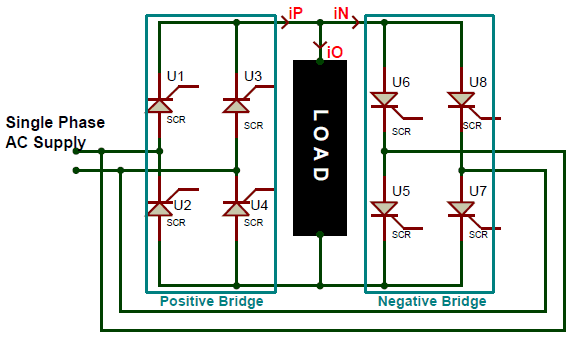All the gate terminals of the SCR’s will be connected to a control circuit which is not shown in the circuit above. This control circuit will be responsible for triggering the SCRs. To understand the working of the circuit let us assume that he input AC supply is of 50Hz frequency and the Load to be a pure resistive load and the firing angle of the SCR (α) to be 0°. Since the firing angle is at 0° the SCR when turned on will act like a diode in forward direction and when turned off will act like a diode in reverse direction. Let us analyse the wave form below to understand how frequency is stepped down using a CCV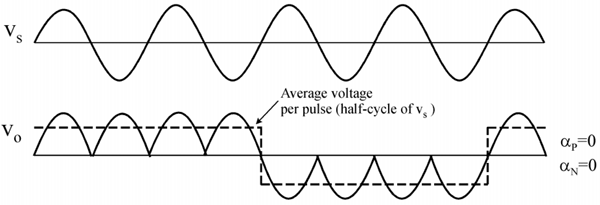The waveform of the supply voltage frequency is denoted by Vs and the wave form of the output voltage frequency is denoted by Vo. Here we are trying to convert the supply voltage frequency to 1/4th of its value. So to do that for the first two cycles of the supply voltage we will use the positive Bridge rectifier and for the following next two cycles we will use the negative bridge rectifier. Thus we have four positive pulses in the positive region and then four in the negative region as shown in the output frequency waveform Vo. The current waveform for this circuit will be the same as voltage waveform since the load is assumed to be purely resistive. Although the magnitude of the waveform will change based on the value of resistance of the load.

The output frequency is represented using the dotted line on the Vo waveform, since it changes polarity only for every two cycles of the input waveform the output frequency with 1/4th of the input frequency, in our case for an input frequency of 50Hz the output frequency will be (1/4 * 50) around 12.5Hz. This output frequency can be controlled by varying the triggering mechanism in the control circuit.

Three Phase to Single Phase Cycloconverters:

The Three Phase to Single Phase CCV is also similar to the Single Phase to Single Phase CCV, but in here the input voltage is a 3 Phase supply and the output voltage is a Single Phase supply with variable frequency. The circuit also looks very similar except we will need 6 SCR in each set of Rectifier since we have to rectify the 3 Phase AC voltage.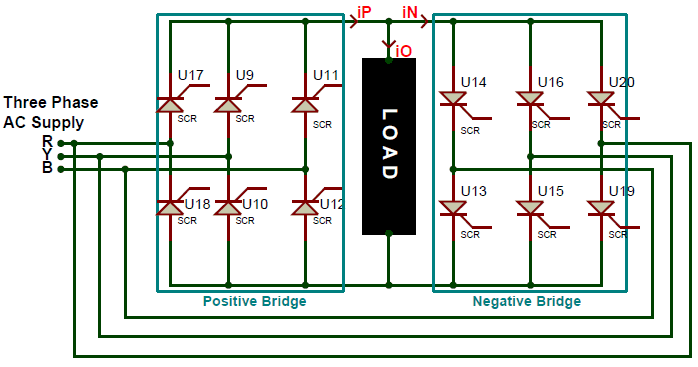Again the gate terminals of the SCR will be connected to the control circuit for triggering them and the same assumptions are made again to understand the working easily. Also there are two kinds of Three Phase to Single Phase CCVs , the first type will have a half wave rectifier for both Positive and Negative Bridge and the second type will have a full-wave rectifier as shown above. The first type is not used often because of its poor efficiency. Also in a full-wave type both the bridge rectifiers can generate voltages in both the polarity, but the positive converter can supply current (source) only in the positive direction and the negative converter can drain current only in negative direction. This allows the CCV to operate in four Quadrants. These four quadrants are (+V, +i) and (-V, -i) in rectification mode and (+V, -i) and (-V,-i) in inversion mode.

Three Phase to Three Phase Cycloconverters:

The Three Phase to Three Phase CCV are the most used ones since they can drive Three Phase loads like motors directly. The Load for a Three Phase CCV will normally be a Three Phase Star connected load like the stator winding of a Motor. This converters take in Three Phase AC voltage with fixed frequency as input and provides Three Phase AC voltage with Variable frequency.

There are two types of Three Phase CCV, the one which has half wave converter and other with full wave converter. The Half wave converter model is also called as 18-thyristor Cycloconverters or 3-pulse Cycloconverters. The full wave converter is called as 6-pulse Cycloconverters or 36-thyristor Cycloconverters. A 3-pulse Cycloconverter is shown in the picture below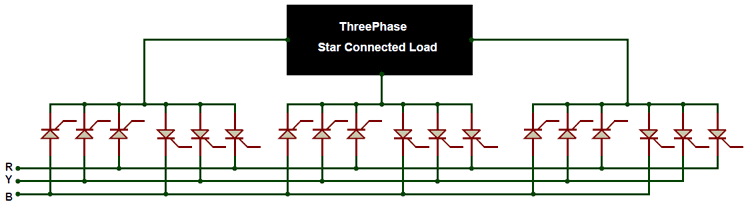Here we have six sets of Rectifiers of which two is allocated for each phase. The working of this CCV is similar to single phase CCV except here the rectifiers can rectify only half the wave and the same happens for all the three phases

Applications of Cycloconverter:

Cycloconverters have a large set of industrial application, the following are the few

• Grinding Mills
• Heavy Washing Machines
• Mine Winders
• HVDC Power lines
• Aircraft Power supply
• SVG (Static VAR Generators)
• Ship Propulsion system